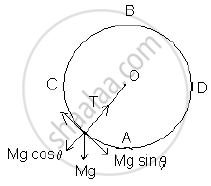# A Particle of Mass M, Just Completes the Vertical Circular Motion. Derive The Expression for the Difference In Tensions at the Highest and the Lowest Points. - Physics

A particle of mass m, just completes the vertical circular motion. Derive the expression for the difference in tensions at the highest and the lowest points.

#### Solution

Consider a body of mass ‘M’ moving in a vertical circle of radius ‘R’. At any position P, the forces are acting body are weight Mg vertically downward and tension ‘T’ towards centre.  Centripetal force FC = T - Mg cosθ

T = FC +Mg cos θThe body is moving such that it can move in a vertical circle.

At position B :  theta = 180^@ , cos theta = - 1 . T_B = F_C – M g

therefore T_B=(MV_B^2)/R -Mg

therefore T_B=(MgR)/R-Mg

therefore T_B=0

At position A : theta = 0^@ , cos theta = 1 , T_B = F_C – M g

T_A = F_C + M g

therefore T_A =(MV_A^2)/R+Mg

therefore T_A=(5MgR)/R+Mg

therefore T_A=6Mg

Difference in tension TB - TA = 6 Mg

Concept: Dynamics of Uniform Circular Motion - Centripetal Force
Is there an error in this question or solution?